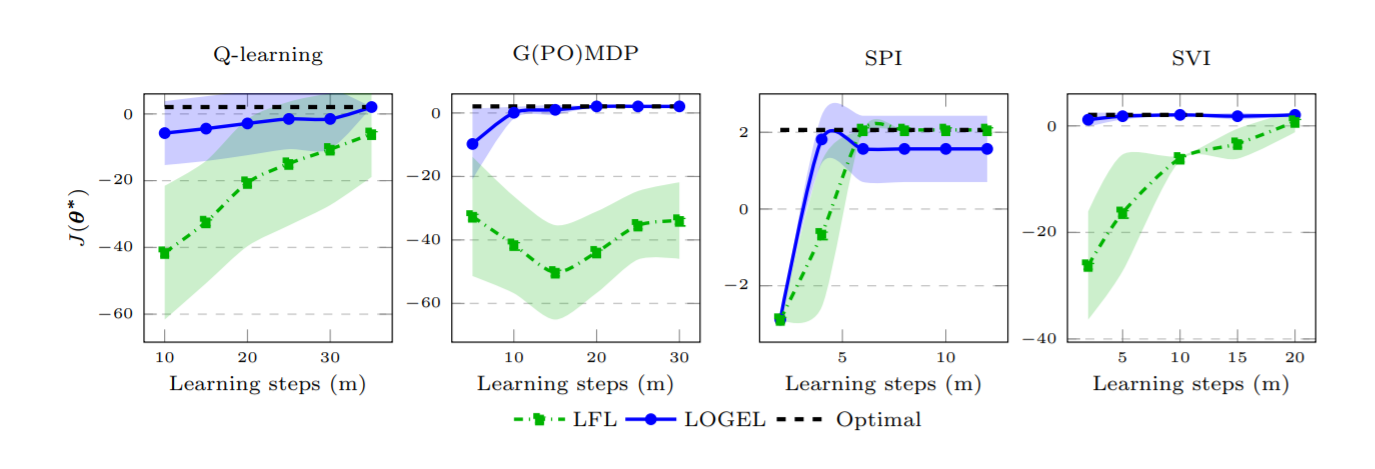# Inverse Reinforcement Learning from a Gradient-based Learner

While searching for gradient based approaches in Inverse Reinforcement Learning, I came across the recent contribution of Ramponi et al. (2020). Here is a short high level summary of the article.

The work of Ramponi et al. (2020) is motivated by the consideration that in many inverse reinforcement learning applications we have access both to the expert’s near-optimal behavior, and its learning process. They propose a new algorithm to recover the reward function of the expert, given a sequence of policies produced during learning.

This approach turns out to be particularly helpful in multi-agent environments in which an agent has to decide whether to cooperate or compete by inferring an unknown reward function that other agents are learning before actually becoming “experts”. Furthermore, by observing the learner behaviors in a variety of situations, it is easier for the observer to generalize the expert’s policy to unobserved situations.

The authors claim that the human learning process is similar to a gradient-based method and extend the work of Jacq et al. (2019) accounting for the non-monotonic learning process.

Preliminaries:

• Markov Decision Process
• Inverse Reinforcement Learning

The Learning from a Learner (LfL) formalism involves two agents:

• A learner which is learning a task defined by the reward function RwL
• An observer which wants to infer the learner’s reward function.

The learner is a RL agent which is learning a policy pi in order to maximize its discounted expected return. The observer perceives the sequence of learner’s policy parameters and a dataset of trajectories for each policy, its goal is to recover the reward function RwL that explains the learner’s policy for all the learner’s updates.

Ramponi et al. assumes that the learner is optimizing the expected discounted return using gradient descent. They initially simplify the problem by giving the observer complete information about the learner’s policy parameters, the associated gradients of the feature expectations, and the learning rates. Then, they replace the exact knowledge of the gradients with estimates built on a set of demonstrations for each learner’s policy. Finally, they introduce the LOGEL algorithm (Learning Observing  Gradient not-Expert Learner) which does not require as input the policy parameters and the learning rates.

In the exact gradient condition, the observer has to find the reward function Rw such that the improvements of the learner are explainable by the gradient-based learning rule.

where

is the Jacobian matrix of the feature expectations w.r.t. the policy parameters theta.

This implies that the observer has to minimize the difference between the expected and the observed features.

In the approximate gradient condition, the observer has no access to the Jacobian matrix but has to estimate it using the dataset D and policy gradient estimator, such as REINFORCE or G(PO)MDP. The observer compares the learner’s weights with the recovered weights for the underlying finite number of learning improvements m (the number of policy improvements steps of the learner is finite as the learner will eventually achieve optimal policy).

In the realistic condition, the observer has to infer the policy parameters, the learning rates and the reward weights. The inference problem is divided into two steps: recovering the policy parameters, and recovering the learning rates and rewards weights. The first step consists in recovering the learner’s policy parameters and generating a dataset of trajectories through behavioral cloning. The second step regards estimating the learning rates by using an optimization method named alternate block-coordinate descent.

The LOGEL algorithm is summarized below:

Where (9) and (5) are respectively:

LOGEL is evaluated by comparing the performance of the algorithm with the state-of-the-art baseline of Learning From a Learner (LfL): a discrete gridworld navigation task, and two MuJoCo continuous environments.

The results of the first set of experiments show that the bias component does not affect the correctness of the observer’s recovered weights.By comparing the performance of LOGEL and LfL in four different learners, the authors notice that LOGEL succeeds in recovering the learner’s reward weights even with learner algorithms other than gradient-based ones, while LfL does not recover the reward weights of the G(PO)MDP and needs more learning steps than LOGEL to learn the rewards wrights when Qlearning learner and Soft policy improvement (SVI) are observed.

For the second set of experiments the learner is trained with Policy Proximal Optimization (PPO) with 16 parallel agents for each learning step. Then they use LOGEL and LfL to recover the reward parameters.

The results show that LOGEL identifies a good reward function in both environments, with a slower recovery in the Reacher environment, while LfL fails to recover an effective reward function for the Hopper environment.

This research has been presented at the Conference on Neural Information Processing Systems (NeurIPS 2020). For further details, take a look at the full paper!